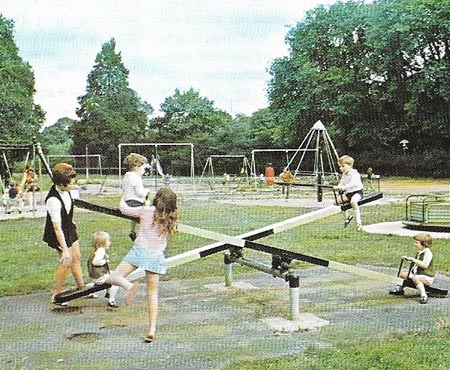A# momentFig 1. The principle of moments is demonstrated by a children's teeter-totter (see-saw).Fig 2. The law of moments

A moment is a tendency to cause rotation about a point or axis, i.e., the turning effect of a force. It is equal to the product of the force F and the perpendicular distance r between the point of axis of rotation (known as the fulcrum) and the line of action of the force, that is

T = Fr

Consider another example. If you push on a door as far away from the hinge as possible, it is very easy to push it open. Pushing nearer to the hinge, though, requires a greater effort. This is because a small force some distance away from the fulcrum can have the same moment as a large force near to the fulcrum.

## Clockwise and anticlockwise moments

The turning effect of a force which tends to turn an object in a clockwise direction is called a clockwise moment and the turning effect of an opposing force is called an anticlockwise moment. When the two moments exactly balance each other then the object is said to be in equilibrium. The simplest way of achieving equilibrium is to use two opposing moments of equal size, but it is quite possible to have one large moment balanced by several smaller moments (Fig 2). Here the sum of the smaller moments is equal in size to the larger moment. Equilibrium can also ne attained by making several opposing moments balance each other. For the object to be in equilibrium the sum of the clockwise moments must equal the sum of the anticlockwise moments. This is known as the law of moments.

Values of moment are given a +/- value depending on whether they tend to produce clockwise or anticlockwise rotation. The international standard (S.I.) unit of moment is the newton·meter. The dimensions of moment are [M ] [L ] 2 [T ]–2.